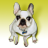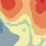# How predicted values are calculated in Geo-statistical Analyst?

436
2
10-25-2013 08:04 AMNew Contributor
Hi,

As you know the last step in Geostatistical Analyst is cross validation.
My question is that, how the predicted value column has been calculated. (attached figure).

[ATTACH=CONFIG]28611[/ATTACH]

My understanding is that Kriging is the exact value interpolation model. This means that the predicted value for the entered data point should be the same as measured one. which results to error equal to zero.

I am not sure how the cross validation has been done in GA. Is it based on some sort of leave one out method?

I would appreciate if you guys could shed some light on this for me.

Thanks

Ehsan
Tags (3)
1 Solution

Accepted SolutionsbyEsri Regular Contributor
Yes, it's a "leave one out" method.  The software throws out a single point, then uses the remaining points to predict back to that location.  It cycles through the entire dataset and calculates lots of diagnostics.

Also, kriging is an exact interpolation method if there is no nugget effect.  You can also force it to be an exact interpolator by turning off measurement error.
2 RepliesbyEsri Regular Contributor
Yes, it's a "leave one out" method.  The software throws out a single point, then uses the remaining points to predict back to that location.  It cycles through the entire dataset and calculates lots of diagnostics.

Also, kriging is an exact interpolation method if there is no nugget effect.  You can also force it to be an exact interpolator by turning off measurement error.New Contributor